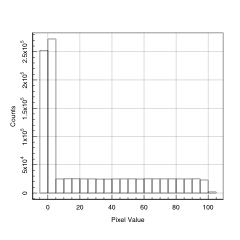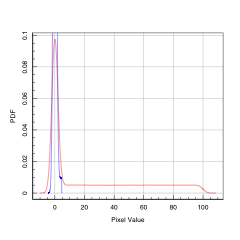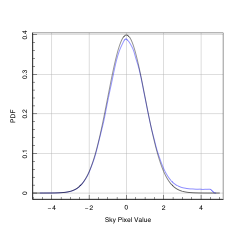# ProFound: Sky Shenanigans

#### 2018-07-31

library(devtools)
install_github('asgr/ProFound')
install_github('ICRAR/ProFit')

Set global evaluate (basically TRUE for GitHub version and FALSE for CRAN):

evalglobal=FALSE

First load the libraries we need:

library(ProFound)
library(ProFit)
library(LaplacesDemon)

## How the Sky is Estimated in ProFound

The sky is estimated mainly via the use of the profoundMakeSkyMap function. This basically does the following:

1. Divide the image into regions based on the requested box car and grid sampling, by default the grid sampling inherits the box car size, meaning each pixels used in every sampling region are unique and all pixels are used
2. Each sub region is analysed separately in a large loop:
1. The masked pixels are removed from analysis, leaving a vector of fiducial sky pixels ($$sky_{fid-pix}$$)
2. Then the following is computed iteratively (either until the clipping is converged, or after 5 iterations):
1. The sky value is estimated as $$sky=median(sky_{fid-pix})$$
2. The dynamic sigma clip level is estimated to be $$\sigma_{clip}=Q_{norm}(1-2/N_{sky})$$
3. The standard deviation of the sky pixels is estimated as $$sky_{RMS}=Quantile(sky_{fid-pix},0.5) - Quantile(sky_{fid-pix}, 0.159)$$
4. The plausible sky pixels are dynamically sigma clipped such that pixels satisfying $$sky_{fid-pix} > sky + sky_{RMS} \sigma_{clip} \lor sky_{fid-pix} < sky - sky_{RMS} \sigma_{clip}$$ are removed
5. The vector of $$sky_{fid-pix}$$ is updated and these new fiducial sky pixels are used for the next iteration
3. Once convergence has been achieved the final computed $$sky$$ and $$sky_{RMS}$$ values are returned for the region under consideration
3. With all regions having a unique estimate of the $$sky$$ and $$sky_{RMS}$$ a bilinear or bicubic interpolation scheme is used to calculate plausible $$sky$$ and $$sky_{RMS}$$ values for all pixels
4. The $$sky$$ and $$sky_{RMS}$$ images are returned to the user or higher level function in a list

## Testing with Toy Data

We can easily create some toy sky with positive flux added in:

set.seed(666)
sky=c(rnorm(5e5,mean=0,sd=1),(rnorm(5e5,mean=0,sd=1)+runif(5e5,0,100)))
maghist(sky, xlab='Pixel Value', ylab='Counts', grid=TRUE)
##  "Summary of used sample:"
##      Min.   1st Qu.    Median      Mean   3rd Qu.      Max.
##  -4.62325  -0.01142   2.04147  25.01887  50.09346 103.34704
##  "sd / 1-sig / 2-sig range:"
##  32.31309 34.40871 48.57714
##  "Using 1000000 out of 1000000"From the text output of maghist we can see that the raw properties output would make very poor estimates of the sky. To do better we need to clip out non-Normal pixels. Luckily we already have access to a function that does exactly this - magclip.

We can run magclip with the estimate set to both (the default) and lo:

sky_clip_both=magclip(sky)
sky_clip_lo=magclip(sky, estimate='lo')

Since we know the fake sources will have positive flux we would expect estimate=lo to work better:

magplot(density(sky_clip_both$x), col='red', grid=TRUE, xlab='Pixel Value', ylab='PDF') lines(density(sky_clip_lo$x), col='blue')Now we can check how good our sky using the lo estimate is, where the black density line is the true sky we want to get back:

magplot(density(sky_clip_lo$x), col='blue', grid=TRUE, xlab='Sky Pixel Value', ylab='PDF') lines(seq(-5,5,len=1e3), dnorm(seq(-5,5,len=1e3)), col='black', grid=TRUE) ## Warning in plot.xy(xy.coords(x, y), type = type, ...): "grid" is not a ## graphical parameterNot bad! The actual sky estimates we end up with are: median(sky_clip_lo$x)
##  0.04500995
diff(quantile(sky_clip_lo$x, pnorm(c(-1,0)))) ## 50% ## 1.019303 Where ideally we want to answer to be 0 and 1. We could instead use the mean and standard-deviation, but these tend to be less robust to contamination: mean(sky_clip_lo$x)
##  0.09501092
sd(sky_clip_lo$x) ##  1.12069 Both of these estimates are notably higher than using the more robust median and qunatile estimates. The main ProFound sky estimation routines eventually end up using magclip to do the clipping, in fact they call each other like this: profoundMakeSkyMap (make a per pixel image) -profoundMarkSkyGrid (make a coarse sky grid) --profoundSkyEstLoc (estimate sky and sky_RMS values in a coarse region) ---magclip (dynamically clip pixels within a coarse region) In practice we also parse profoundMakeSkyMap (and all lower functions) a bas pixel mask and/or a binary object mask, so we remove from consideration all of the clearly non-sky pixels before even attempting to do the clipping on what is left. ## Testing with More Realistic Data We can do a more realistic test by using the sky estimate maps from the test VIKING Z-band data we include with ProFound: image=readFITS(system.file("extdata", 'VIKING/mystery_VIKING_Z.fits', package="ProFound")) profound=profoundProFound(image, skycut=1, magzero=30, plot=TRUE) From this we get a reasonable sky and sky-RMS map: magimage(profound$sky)
magimage(profound$skyRMS) maghist(profound$sky, xlab='Pixel Value', ylab='Counts', grid=TRUE)
maghist(profound$skyRMS, xlab='Pixel Value', ylab='Counts', grid=TRUE) You can see in the sky-RMS histograms that there are two distinct depths in the test data. This is due to data being stacked to make the final image. We can now make a noise free simulated image with a mixture of stars and galaxies (the extraction found 67 sources, and we use ProFound properties where appropriate): ExamplePSF=profitMakeGaussianPSF(fwhm=5) ExamplePSF=ExamplePSF/sum(ExamplePSF) model_test=list( sersic=list( xcen=runif(67,0,356), ycen=runif(67,0,356), mag=profound$segstats$mag, re=profound$segstats$R50, nser=runif(67,0.5,4), ang=runif(67,0,180), axrat=runif(67,0.3,1), box=rep(0,67) ) ) im_test<-profitMakeModel(modellist=model_test, psf=ExamplePSF, dim=c(356,356), magzero = 30)$z

Let’s take a look:

magimage(im_test)

We can now add back in the sky and sky-RMS we just calculated:

im_test_noise=im_test+rnorm(356^2, mean=profound$sky, sd=profound$skyRMS)

Let’s take a look:

magimage(im_test_noise)

We can now run ProFound on this simualted data:

profound_resim=profoundProFound(im_test_noise, skycut=1, magzero=30, plot=TRUE)

So finally we can check our new sky estimates:

magimage(profound$sky) magimage(profound$skyRMS)

magimage(profound_resim$sky) magimage(profound_resim$skyRMS)

magimage(profound$sky-profound_resim$sky)
magimage(profound$skyRMS-profound_resim$skyRMS)

It is perhaps more useful to look at the histograms directly:

maghist(profound$sky, xlab='Pixel Value', ylab='Counts', grid=TRUE) maghist(profound$skyRMS, xlab='Pixel Value', ylab='Counts', grid=TRUE)

maghist(profound_resim$sky, xlab='Pixel Value', ylab='Counts', grid=TRUE) maghist(profound_resim$skyRMS, xlab='Pixel Value', ylab='Counts', grid=TRUE)

maghist(profound$sky-profound_resim$sky, xlab='Pixel Value', ylab='Counts', grid=TRUE)
maghist(profound$skyRMS-profound_resim$skyRMS, xlab='Pixel Value', ylab='Counts', grid=TRUE)

Broadly we can see many of the sky features are being found in the same places on the image.

The simplest metric to decide whether we are, on average, doing the right thing is to compare the standard deviation in the input sky to that obtained from the input-estimated sky:

sd(profound$sky) sd(profound$sky-profound_resim$sky) sd(profound$skyRMS)
sd(profound$skyRMS-profound_resim$skyRMS)

We can see there is a large reduction in the absolute sky variance, and a small reduction in the sky-RMS variance. The take-home here is that ProFound is at least bringing the sky and sky-RMS closer to the intrinsic values (i.e. better than doing nothing or using a fixed estimator), but it is not perfect.

## Bias as a Function of Number of Sky vs. Object pixels

A good question is how contaminated the sky pixels can be (i.e. non-masked and non-object pixels) for us to still get a decent estimate of the true sky.

We can do this experiment roughly by varying the number of pixels with object flux in our toy sky model from earlier:

contam=seq(1e5, 2e6, by=1e5)
N=length(contam)
output=cbind(contam,rep(0,N), rep(0,N), rep(0,N), rep(0,N))
for(i in 1:N){
sky=c(rnorm(5e5,mean=0,sd=1),(rnorm(contam[i],mean=0,sd=1)+runif(contam[i],0,100)))
sky_clip_lo=magclip(sky, estimate='lo')
output[i,2]=as.numeric(median(sky_clip_lo$x)) output[i,3]=as.numeric(diff(quantile(sky_clip_lo$x, pnorm(c(-1,0)))))
output[i,4]=as.numeric(mean(sky_clip_lo$x)) output[i,5]=as.numeric(sd(sky_clip_lo$x))
}

We can plot how this behaves:

magplot(1-output[,1]/(5e5+output[,1]),output[,2], log='y', grid=TRUE, xlab='Nsky/Ntotal', ylab='(Sky Estimate) - (Sky Input)', type='l', col='red')
lines(1-output[,1]/(5e5+output[,1]),output[,4], col='blue')
legend('topright', legend=c('Median','Mean'), lty=1, col=c('red','blue'))

magplot(1-output[,1]/(5e5+output[,1]),output[,3]-1, log='y', grid=TRUE, xlab='Nsky/Ntotal', ylab='(Sky-RMS Estimate) - (Sky-RMS Input)', type='l', col='red')
lines(1-output[,1]/(5e5+output[,1]),output[,5]-1, col='blue')
legend('topright', legend=c('Quantile Range','Standard-Deviation'), lty=1, col=c('red','blue'))

We can see that both the sky and sky-RMS estimates behave best when the contamination level is small (i.e. they are pretty unbiased when the sky to object ration is 5:1). From this point they become gradually more biased (always positively), and then they fail spectacularly when the sky to object ration is 5:8 or above (i.e. less than 40% of pixels are sky pixels).

The example above is only for a simple toy model, but it gives you some idea that you want comfortably more than half of the pixels to be real sky pixels in general. Obviously using the bad pixel mask and the objects mask helps a lot, but in detail very few pixels are pure sky pixels because there are always increasingly faint (sub surface brightness limit) sources that bias the flux positively and in a non-uniform manner. This is similar to saying that all images are confused, you just cannot always tell by looking at them. This fact is more obvious when you consider the earlier simulated image data before and after we add realistic sky noise. Those broad low-surface brightness wings are still there in the noisy image, they are just well below the applied noise level. However, those low surface brightness features are still able to bias our estimated sky away from the intrinsic values we would like to know.

The difference between the sky level estimators (mean, median or mode) and sky-RMS level estimator (quantile vs. standard-deviation) is an interesting point to consider. In the toy situation described it is easy to convince yourself that you would want to use the median for the sky and the quantile for the sky-RMS, since these are both systematically nearer to the specified ‘intrinsic’ sky. However, what really matters is the origin of the positively biased flux that skews the distribution. There are a number of processes that generate the ‘sky’ and contribute to the extended area signal in a typical CCD image, in roughly descending order of importance:

1. The actual night sky caused by Earth’s atmosphere glowing. Can be quite spatially and temporally variable (in the NIR, distant artificial lights turning on and off etc)
2. Flux scattered around the image due to telescope optics causing very broad ~Lorentzian wings
3. Scattering of astronomical light by the Earth’s atmosphere, usually at very small scales (close to Gaussian usually)
4. Intrinsically broad features caused by the Milky-Way foreground
5. Intrinsically broad wings caused by extra-galactic sources (low surface brightness wings of galaxies)
6. Undetectable faint sources, can be structured (Milky-Way stars) or effectively uniform in distribution (high redshift galaxies)

The question is then: which of these do you want to remove? The answer clearly depends on what sources you are trying to extract photometry for. If you are doing stellar photometry of a bright star then you almost certainly want to remove 1/4/5/6, i.e. you actually want to model the light from the star that has been scattered by the atmosphere and the telescope (assuming it is the dominant contribution close to the star being modelled). If you want to profile a faint galaxy then you probably need to remove 1/2 (assuming it mostly caused by other source)/3 (assuming it mostly caused by other source)/4/6, i.e. you want to keep the faint wings of your target galaxy intact. There is not a trivially right answer, but ProFound does offer a few routes to compute these different types of sky. The basic message is you might prefer a median type sky or a mean type sky, depending on your use case. If your source sits on top of the sky (whatever makes it) then you probably want to use the more biased mean estimator. If your source is the dominant part of the observable background (e.g. when profiling the faint wings of galaxies) then you might prefer the less biased median estimator.

An unresolved issue is whether you can extract better models by using ProFit to model the background for a given source. The three obvious options are:

• Use ProFound sky subtraction on the image and do not fit a sky background in ProFit
• Use ProFound sky subtraction on the image and also fit a sky background in ProFit
• Do not use the ProFound sky subtraction on the image and only fit a sky background in ProFit

For pragmatic reasons of needing to remove complex sky that cannot be fully generatively modelled with , options 1 or 2 are likely to be the best strategy with the majority of use cases.

## ProFound Sky Options

Below we will run the ProFound sky routine with and without sky clipping and using the three different estimators (mean, median, mode):

profound_median_clip=profoundProFound(image, skycut=1.0, magzero=30, skytype='median', doclip=TRUE) #The default mode
profound_median_noclip=profoundProFound(image, skycut=1.0, magzero=30, skytype='median', doclip=FALSE)
profound_mean_clip=profoundProFound(image, skycut=1.0, magzero=30, skytype='mean', doclip=TRUE)
profound_mean_noclip=profoundProFound(image, skycut=1.0, magzero=30, skytype='mean', doclip=FALSE)
profound_mode_clip=profoundProFound(image, skycut=1.0, magzero=30, skytype='mode', doclip=TRUE)
profound_mode_noclip=profoundProFound(image, skycut=1.0, magzero=30, skytype='mode', doclip=FALSE)

The difference between the clipped and non-clipped runs are tiny (meaning we have found pretty much all of the sources):

maghist(profound_median_clip$sky-profound_median_noclip$sky, grid=TRUE)
maghist(profound_mean_clip$sky-profound_mean_noclip$sky, grid=TRUE)
maghist(profound_mode_clip$sky-profound_mode_noclip$sky, grid=TRUE)

The differences between the different estimators are larger:

maghist(profound_mean_clip$sky-profound_median_clip$sky, grid=TRUE)
maghist(profound_mode_clip$sky-profound_median_clip$sky, grid=TRUE)
maghist(profound_mean_clip$sky-profound_mode_clip$sky, grid=TRUE)

The median and the mode are the most similar, and for most people the choice will come down to using one of these two. We can check the impact this choice might have on the photometry:

magplot(profound_median_clip$segstats$mag, profound_median_clip$segstats$mag-profound_mean_clip$segstats$mag, ylim=c(-0.1,0.1), xlab='Median mag', ylab='(Median mag) - (Mean mag)', grid=TRUE)
lines(magrun(profound_median_clip$segstats$mag, profound_median_clip$segstats$mag-profound_mean_clip$segstats$mag), col='red')

So even down to our detection limit the difference tends to be a few hundredths of a mag on average, with the average effect that subtracting a median sky leaves more flux in the image (on average) given us brighter measured magnitudes. For this reason it is probably a good check to always run ProFound with both types of sky and check there are not regions with pathelogically weird differences.

## Improving Our Sky Map with FFTs

By doing ProFound source detection on the FFT itself it tells us if there are significant sources of a certain common scale (usually small) still in the image to extract. We can improve the sky using profoundSkySplitFFT.

profound=profoundProFound(image, type="bicubic")
newsky=profoundSkySplitFFT(image$imDat, objects=profound$objects_redo, sky=profound$sky, skyRMS=profound$skyRMS)

We can check the old versus new sky:

magimage(profound$sky) magimage(newsky$sky)

We can have a look at the original image, old sky subtraction and new sky subtraction (pretty subtle!):

magimage(image$imDat) magimage(image$imDat-profound$sky) magimage(image$imDat-newsky$sky) Be warned, you need a reasonable estimate of the sky and objects before running profoundSkySplitFFT. If we run on the original image that even the high/low k modes look very odd: magimage(profoundSkySplitFFT(image$imDat)$sky_lo) magimage(profoundSkySplitFFT(image$imDat)\$sky_hi)

## Doing Better

I (ASGR) spent a bit of time looking at whether you can do better by attempting to fit a true generative model to the sky. Even when the model is exactly the type used to generate the sky (which will not true for real data of course, the toy setup here is only crudely realistic) there is such a degeneracy between betweent the sky level and the mode of the Normal sky / start of the uniform object distribution, that you rarely do better than the clipped estimate used in ProFound currently. By using medians and quantiles we are also more robust to Lorentzian wings of weirdness, bright star artefacts, and cosmic ray effects (which can even created negative signals depending on how the sky pedestal is implemented).

All this said, I am all ears as to how we might be able to estimate the sky better. Any estimator must be pragmatic (be able to cope with the fact that real data is not perfect, not in detail Normal, etc), so I hope you can appreciate that this is not a trivial task.